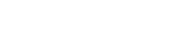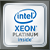Data Science Solution Center

# Accelerating Linear Models for Machine Learning

Linear Regression Has Never Been Faster

By Victoriya Fedotova, Machine Learning Engineer at Intel Corporation

If you have ever used Python and scikit-learn to build machine learning (ML) models from large data sets, you may have also wished that you could make these computations go faster. What if I told you that altering a single line of code could accelerate your ML computations? What if I also told you that getting faster results doesn’t require specialized hardware?

In this article, I will teach you how to train ridge regression models using a version of scikit-learn that is optimized for Intel CPUs, then compare the performance and accuracy of these models trained with the vanilla scikit-learn library. This article continues our series on accelerated ML algorithms.

## A Practical Example of Linear Regression

Linear regression, a special case of ridge regression, has a lot of applications. For my comparisons, I’m going to use the well-known House Sales in King County, USA data set from Kaggle. This data set is used to predict house prices based on one year of King County sales data.

This data set has 21,613 rows and 21 columns. Each row represents a house that was sold in King County between May 2014 and May 2015. The first column contains a unique identifier for the sale, the second column contains the date the house was sold, and the third column contains the sale price, which is also the target variable. Columns 4 to 21 contain various numerical characteristics of the house, such as the number of bedrooms, square footage, the year when the house was built, zip code, etc. I am going to build a ridge regression model that predicts the price of the house based on the data in columns 4 to 21.

In theory, the coefficients of the linear regression model should have the lowest residual sum of squares (RSS). In practice, the model with the lowest RSS is not always the best. Linear regression can produce inaccurate models if input data suffers from multicollinearity. Ridge regression can give more reliable estimates in this case.

## Solving a Regression Problem with scikit-learn

Let’s see how to build a model with sklearn.linear_model.Ridge. The program below trains a ridge regression model on 80% of the rows from the House Sales dataset, then uses the other 20% to test the model’s accuracy.

`import numpy as npimport pandas as pdfrom sklearn import config_contextfrom sklearn.linear_model import Ridgefrom sklearn.model_selection import train_test_split# Load the data from a text file into pandas DataFrames.# The third column contains the responses. Load it into df_y.# Columns after the fourth contain features. Load them into df_X.infile = "data/kc_house_data.csv"df_X = pd.read_csv(infile, usecols=range(3, 20))df_y = pd.read_csv(infile, usecols=)# Split the data into training and testing subsets.# Use 80% of the rows to train the regression model.# Use the remaining 20% to test the model.X_train_np, X_test_np, y_train_np, y_test_np =   \    train_test_split(df_X, df_y, train_size=0.8, \                      random_state=7777777)# Convert the data into pandas DataFrames for convenience:X_train = pd.DataFrame(X_train_np)y_train = pd.DataFrame(y_train_np)X_test = pd.DataFrame(X_test_np)y_test = pd.DataFrame(y_test_np)# Construct a ridge regression algorithm object:alg = Ridge(alpha=1.0)# Train a ridge regression model:model = alg.fit(X_train, y_train)# Check the model's accuracy on a test set:y_pred = model.predict(X_test)MSE = ((y_test.values - y_pred)**2).sum()RMSD = np.sqrt(MSE/y_test.size)R2 = alg.score(X_test, y_test)`

The resulting equals 0.69, meaning that our model describes 69% of variance in the data. See the Appendix for details on the quality of the trained models.

## Intel-Optimized scikit-learn

Even though ridge regression is quite fast in terms of training and prediction time, you usually need to perform multiple training experiments to tune the hyperparameters. You might also want to experiment with feature selection (i.e., evaluate the model on various subsets of features) to get better prediction accuracy. Since one round of training can take several minutes on large data sets, which can quickly add up if your task requires multiple rounds of training, the performance of linear model training is critical.

Intel Distribution for Python is a drop-in replacement for the native Python installation, but it is optimized for Intel architectures. It works seamlessly with the packages available for installation through common channels such as conda and pip. The scikit-learn in Intel Distribution for Python has the same set of algorithms and the APIs as Continuum’s scikit-learn, so no code changes are required to get a performance boost for ML algorithms.

Also, with daal4py, a Python interface to Intel oneAPI Data Analytics Library (oneDAL), it is possible to improve the performance of scikit-learn even further. daal4py provides configurable ML kernels, some of which support streaming input data and can easily be scaled out to clusters of workstations.

## How to Configure scikit-learn with daal4py

There are several ways to install Intel Distribution for Python. Follow these instructions to install it with conda.

The following command installs daal4py into an ‘idp’ conda environment:

conda install -n idp daal4py

Dynamic patching of scikit-learn is required to use daal4py as the underlying solver. You can enable patching without modifying your application. Just use the following command-line flag:

python -m daal4py my_app.py

Patching also can be enabled within your application:

`import daal4py.sklearndaal4py.sklearn.patch_sklearn()`

To undo the patch, run:

daal4py.sklearn.unpatch_sklearn()

Applying the patch impacts the following scikit-learn algorithms:

This list will continue to grow in the next releases of Intel Distribution for Python.

## Performance Comparison

To compare performance of the vanilla scikit-learn and Intel-optimized scikit-learn, we used the King County data set plus six artificially generated datasets with varying numbers of samples and features. The latter were generated using the scikit-learn make_regression function:

`X, y = make_regression(n_samples=nrows, n_features=ncols, \                       n_informative=ncols, noise=10.0, bias=10.0, \                       random_state=7777777)X_train = pd.DataFrame(X)y_train = pd.DataFrame(y)`

Figure 1 shows the wall-clock time spent on training a ridge regression model with two different configurations:

• Scikit-learn version 0.22 installed from the default set of conda channels.
• Scikit-learn version 0.21.3 from Intel Distribution for Python optimized with daal4py.

To enable oneDAL optimizations in scikit-learn, we used the -m daal4py command-line option. For performance measurements, we used the Amazon Web Services Elastic Compute Cloud (AWS EC2). We chose the instance that gives best performance:

Amazon states that

C5 instances offer the lowest price per vCPU in the Amazon EC2 family and are ideal for running advanced compute-intensive workloads.

c5.metal instance has the most CPU cores and the latest CPUs among the C5 instances.

See the Configuration section below for hardware details. See the Appendix for details on the quality of trained models.

Figure 1 Ridge regression training time

Figure 1 shows that:

• Ridge regression training is up to 5.49x faster with the Intel-optimized scikit-learn than with vanilla scikit-learn.
• The performance improvement from the Intel-optimized scikit-learn increases with the size of the data set.

## What Makes scikit-learn in Intel Distribution for Python Faster?

For the big data sets, ridge regression spends most of its compute time on matrix multiplication. oneDAL’s implementation of ridge regression relies on the Intel Math Kernel Library (Intel MKL), which is highly-optimized for Intel CPUs. Intel MKL uses Single Instruction Multiple Data (SIMD) vector instructions from the Intel Advanced Vector Extensions 512 (Intel AVX-512) available on 2nd Generation Intel Xeon Scalable processors. Compute-intensive kernels like matrix multiplication benefit significantly from the data parallelism that these instructions provide. Another level of parallelism for matrix multiplication is achieved by splitting matrices into blocks and processing them in parallel using Threading Building Blocks (TBB).

The Intel-optimized version of scikit-learn gives significantly better performance for ridge regression with no loss of model accuracy and little to no code modification. The performance advantages are not limited to just this algorithm. As mentioned previously, the list of optimized ML algorithms continues to grow.

## Configuration

### Hardware

c5.metal AWS EC2 instance: Intel Xeon 8275CL processor, two sockets with 24 cores per socket, 192 GB RAM. OS: Ubuntu 18.04.3 LTS.

Testing date: 04/06/2020

### Software

Vanilla sklearn: Python 3.8.0, scikit-learn 0.22, Pandas 0.25.3.

Intel Distribution for Python scikit-learn: Conda install from intel channel: Python 3.7.4, scikit-learn 0.21.3 optimized with daal4py 2020.0 build py37ha68da19_8, Pandas 0.25.1.

Python and accompanying libraries are the default versions installed by the conda package manager when configuring the respective environments.

### Appendix

Table 1 Root mean squared deviation (RMSD) and the coefficient of determination (R²) for ridge regression models